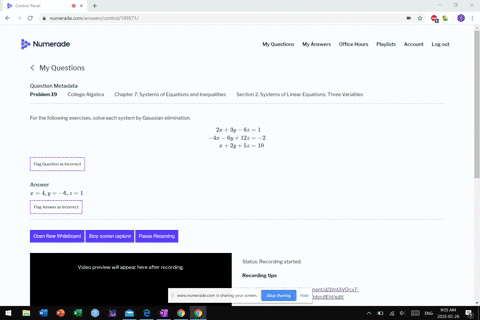Sign up for our free STEM online summer camps starting June 1st!View Summer Courses### For the following exercises, solve each system by…

03:25
MB

Need more help? Fill out this quick form to get professional live tutoring.

Get live tutoring
Problem 18

For the following exercises, solve each system by Gaussian elimination.
\begin{aligned} 5 x-6 y+3 z &=50 \\-x+4 y &=10 \\ 2 x-z &=10 \end{aligned}

$x=-0.05$
$y=0.5$
$z=-0.31$

## Discussion

You must be signed in to discuss.

## Video Transcript

All right. So now we have a system again. Three equations with three unknowns, and we're gonna solve this using Alysia elimination method. Meaning weekend Pretty much. Do anything. We want to make an ad equations together, substitute patients in the equations. Mom, do you think I want to? So, e c e. I think I'm gonna solve, um, this last one z So, um, move is the office adds either both sides and subtract tents to X minus 10 people. See? All right. And now that I have that, I'm gonna take this expression Rizzi substituted. Been here? Yes. I will no longer have disease in the top equation. So I get Come five x minus six. Why? Plus three Time Z, which is now two x minus 10. People's 50. Simplify this. So I'd still get five months. It's why. Plus it's X minus 30 equals 50. And so that needs me with 11 x minus six. Why equals terms? 11 x months. It's wine at 30 of both sides. So I'm gonna combine. That s I'd use this equation. I'm used this equation. I need to involve this separation to someone. Just go ahead and combine the answer to my its last answer Over here, Mama Maria's combined that. So get negative X plus for why he wants home. So do anything but not that system of two equations and hornets. So multiply the bottom equation by 11. Okay, so the top equation stays 11 X minus six. Why people's 80 now the bottom equation is negative. 11 X plus 44. Why equals 110? Add these two equations together. 44 minus six is 38. Why equals 100 lining right? And then 1 90 divided by 38 begins size Wow. Okay, cool the way it was. Five. All right, so now I know why is five months I find X? So let's use this equation here. Get neighbors. Thanks. Plus or charms equals 10. So negative. That's people's track 2010 10. So actually 10. And then finally, divine Z z is gonna be people too. Two times X minus 10 that B 20 minus 10. Which is also so my I'm Lance, would X Why? Z